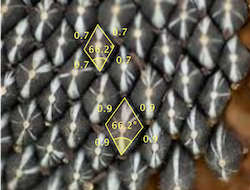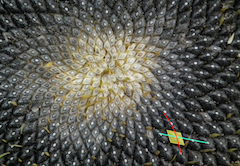# Sunflowers and Special Numbers

This applet simulates the growth and arrangement of seeds in a sunflower head. A look at the image below shows tightly packed rhombuses. The angle of the rhombuses may vary depending on the plant variety, but the structure is the same. The applet uses a rhombus angle of .Figure 1
In nature, when the sunflower head starts to form, all seeds are very small and clustered in the center. As they begin to grow they are pushed away from the center and from one another, on a constant divergence angle . When we measure , most often it equals approximately . In geometry this angle is known as the golden angle. This is the smaller angle formed by two radii in a circle such that the ratio of the length of the larger arc to the length of the smaller arc equals the ratio of the circumference of the circle to the length of the larger arc. *
I. Use the applet below to see how the sunflower head would look for different values of .
• Change angle and notice the difference in the packing of the seeds.
• Find other angles creating tight packing of the seeds.
• Use the input box to enter values for in the form , or where you can choose some rational values of like and some irrational values, for example, sqrt(2), e, phi (for the golden ratio).
• What did you notice? For what values of the seeds are positioned in the most efficient way?
The golden ratio is called sometimes the most irrational number. Very often plants use the golden angle of growth to achieve optimal positioning of the seeds or leafs in order to maximize the exposure to sunlight and to minimize space.
II. Next we will examine the spiral structure of the arrangement of the seeds. If we start from one seed and connect two opposite sides of the rhombus, and then continue this process with the neighboring seeds, we will draw a part of a spiral. Choosing the other pair of opposite sides of the rhombus will form a second spiral in the opposite direction. Figure 2 shows two families of spirals starting from the center, one going to the right and the other one going to the left. We can use the applet to explore these two spirals.Figure 2
Let us number the seeds as they begin to grow and move away from the center. The “first” seed will be at the edge of the disk. Make sure equals the golden angle.
• Click the checkbox “connect every seed”. The line connecting the seeds follows one of the naturally occurring spirals.
• The checkbox “Connect every seed” will reveal another spiral in the opposite direction.
• Change the values of and . For what values we see a smooth line?
• Do you recognize these numbers?
The Fibonacci numbers are the numbers formed from two initial values and , and every next number is defined by adding the previous two numbers in the sequence: These numbers occur in a very natural way in the growth of many plants. The ratio of any two consecutive Fibonacci numbers converges to the golden ratio.
• Clicking the checkboxes “Show All” will display all possible such spirals. How many are the spirals in each direction? Based on the way the spirals are constructed, can you find their number without counting them?
III. Not all plants form their seeds (or leafs) following the golden angle.
• Change the angle of growth to and keep and two consecutive Fibonacci numbers. The connecting lines are not spirals anymore!
• Change the value of and and find two numbers that generate two spirals in opposite directions, following the arrangement of the seeds.
• Do you recognize these numbers?
The Lucas numbers are formed in the same way as the Fibonacci numbers, but with different initial values, i.e.
• Try to explain the occurrence of the Lucas numbers in the natural growth by calculating the ratio between two consecutive Lucas numbers and predicting the limit of this ratio.
• Try other values of to see if there are other possible good growth patterns.
The divergence angle is found in approximately 92% of the plants. Other less frequently observed angles are .  ﻿
• Change the value of and and find two numbers that generate spirals in opposite directions, following the arrangement of the seeds using the above angles.
• What Fibonacci-like sequences describe the spiral arrangements generated by the new divergence angles?
_____________________________________________________________ *Пояснительные выражения объясняют темные мысли. Козьма Прутков  Phyllotaxis: A Systemic Study in Plant Morphogenesis, Roger V. Jean, Cambridge University Press.